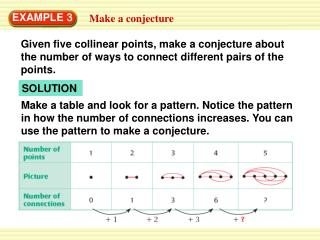DownloadDownload PresentationEXAMPLE 3

# EXAMPLE 3

Télécharger la présentation## EXAMPLE 3

- - - - - - - - - - - - - - - - - - - - - - - - - - - E N D - - - - - - - - - - - - - - - - - - - - - - - - - - -
##### Presentation Transcript

1. EXAMPLE 3 Make a conjecture Given five collinear points, make a conjecture about the number of ways to connect different pairs of the points. SOLUTION Make a table and look for a pattern. Notice the pattern in how the number of connections increases. You can use the pattern to make a conjecture.

2. ANSWER Conjecture: You can connect five collinear points 6 + 4, or 10 different ways. EXAMPLE 3 Make a conjecture

3. = 4 3 = 8 3 = 113 = 17 3 ANSWER Conjecture: The sum of any three consecutive integers is three times the second number. EXAMPLE 4 Make and test a conjecture Numbers such as 3, 4, and 5 are called consecutive integers. Make and test a conjecture about the sum of any three consecutive numbers. SOLUTION STEP 1 Find a pattern using a few groups of small numbers. 3 + 4 + 5 = 12 7 + 8 + 9 = 12 10 + 11+ 12 = 33 16 + 17 + 18 = 51

4. = 101 3 = 0 3 EXAMPLE 4 Make and test a conjecture STEP 1 Test your conjecture using other numbers. For example, test that it works with the groups –1, 0, 1 and 100, 101, 102. 100 + 101 + 102 = 303 –1 + 0 + 1 = 0

5. 3. Suppose you are given seven collinear points. Make a conjecture about the number of ways to connect different pairs of the points. Number of points. 1 2 3 4 5 6 7 . . . . . . . . . . . . . . . . . . . . . . . . . . . . Picture Number of connections 0 1 3 6 10 15 ? +1 +2 +3 +4 +5 +? for Examples 3 and 4 GUIDED PRACTICE

6. ANSWER Conjecture: You can connect seven collinear points 15 + 6, or 21 different ways. for Examples 3 and 4 GUIDED PRACTICE

7. 4. Make and test a conjecture about the sign of the product of any three negative integers. ANSWER Conjecture: The result of the product of three negative number is a negative number. Test: Test conjecture using the negative integer –2, –5 and –4 –2 –5 –4 = –40 for Examples 3 and 4 GUIDED PRACTICE